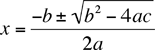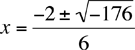My name is AL and my daughter got quite a brain teaser. As a parent I though for sure I’d be able to figure it out… Is this unsolvable??? 2xy + 3x2 - 3y + y3 = 4x + 3 where y=3. 6x + 3x2 + 18 = 4x + 3 After some reorganization I came up with 3x2 + 2x = -15, which through logic appears to be unsolvable… Or x(x + 2/3) = -5 or still x + 2/3 = -5/x So I thought maybe he (the teacher) meant y= -3 Hi Al, You got 3x2 + 2x = -15 or 3x2 + 2x + 15 = 0. At this point I would use the general quadratic equation which is that if ax2 + bx + c = 0 thenwhich in your case givesThis is called a complex number, numbers that involve the square roots of negative numbers. Your daughter might be familiar with these or it may be that the teacher meant y = -3 which gives real number answers as opposed to complex numbers. In that case 3x2 + 2x - 21 = 0 factors as (3x - 7)(x+3) = 0, which yields the two solutions x = 7/3 and x = -3. Penny and Claude